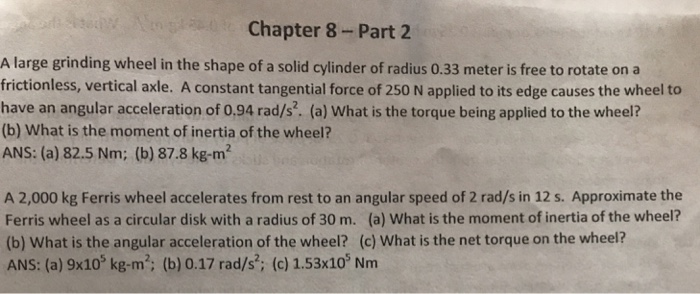Question

# A 2700-kg Ferris wheel accelerates from rest to an angular speed of 0.16 rad/s in 14...

A 2700-kg Ferris wheel accelerates from rest to an angular speed of 0.16 rad/s in 14 s . Approximate the Ferris wheel as a circular disk with a radius of 23 m . What is the net torque on the wheel?

Net Torque = _____ m*N

Solution)

Mass,m=2700 kg

Time, t=14 s

Here, Moment of Inertia, I= 0.5 mr^2= 0.5*2700*23^2

=714150 kg-m^2

Also, angular acceleration, alpha=0.16/14= 0.0114 rad/s^2

Now, we know

Torque, T= I*alpha=714150*0.0114= 8161.7 N=8161700 mN (Ans)

==============

#### Earn Coins

Coins can be redeemed for fabulous gifts.

Similar Homework Help Questions
• ### Chapter 8-Part 2 A large grinding wheel in the shape of a solid cylinder of radius...Chapter 8-Part 2 A large grinding wheel in the shape of a solid cylinder of radius 0.33 meter is free to rotate on a frictionless, vertical axle. A constant tangential force of 250 N applied to its edge causes the wheel to have an angular acceleration of 0,94 rad/s2. (a) What is the torque being applied to the wheel? (b) What is the moment of inertia of the wheel? ANS: (a) 82.5 Nm; (b) 87.8 kg-m2 A 2,000 kg Ferris...

• ### A Ferris wheel rotates at an angular velocity of 0.26 rad/s. Starting from rest, it reaches...

A Ferris wheel rotates at an angular velocity of 0.26 rad/s. Starting from rest, it reaches its operating speed with an average angular acceleration of 0.034 rad/s2. How long does it take the wheel to come up to operating speed?

• ### A giant Ferris wheel is spinning at 70 rad/s. It is switched off and accelerates at the rate of -10 rad/s2

6) A giant Ferris wheel is spinning at 70 rad/s. It is switched off and accelerates at the rate of -10 rad/s2. How many full revolutions will it complete in 3.0 s?9) What is the total initial acceleration of a tether ball on a 2.0 m rope whose angular velocity changes from 13 rad/s to 7.0 rad/s in 15 s?12) A wheel rotates at an angular velocity of 30 rad/s. If an acceleration of 26 rad/s2 is applied to it,...

• ### A merry-go-round accelerates from rest to 0.63 rad/s in 36 s. Assuming the merry-go-round is a...

A merry-go-round accelerates from rest to 0.63 rad/s in 36 s. Assuming the merry-go-round is a uniform disk of radius 8.0 m and mass 3.20×104 kg , calculate the net torque required to accelerate it.

• ### A Ferris wheel undergoes a constant angular acceleration as it starts from rest and builds up...

A Ferris wheel undergoes a constant angular acceleration as it starts from rest and builds up to a final angular speed of 1.9 rad/s while rotating through an angular displacement of 4.9 rad. Find its angular acceleration.

• ### A merry-go-round accelerates from rest to 0.68 rad/s in 24 s. Assuming the merry-go-round is a uniform disk of radius...

A merry-go-round accelerates from rest to 0.68 rad/s in 24 s. Assuming the merry-go-round is a uniform disk of radius 7 m and mass 31000 kg, calculate the net torque required to accelerate it.

• ### A fly wheel with radius 0.300 m starts from rest and accelerates with a constant angular acceleration of 0.600 rad/s2

A fly wheel with radius 0.300 m starts from rest and accelerates with a constant angular acceleration of 0.600 rad/s2. For a point on the rim of the flying wheel, what are the magnitudes of the tangential, radial, and resultant accelerations and velocities after 2.00 seconds?

• ### A Fly wheel with radius 0.300 m starts from rest and accelerates with a constant angular acceleration of 0.600 rad/s2.

A Fly wheel with radius 0.300 m starts from rest and accelerates with a constant angular acceleration of 0.600 rad/s2. For a point on the rim of the flying wheel, what are the magnitudes of the tangential, radial, and resultant accelerations and velocities after 2.00 seconds?

• ### A merry-go-round accelerates from rest to 0.74 rad/s in 33 s. Part A Assuming the merry-go-round...

A merry-go-round accelerates from rest to 0.74 rad/s in 33 s. Part A Assuming the merry-go-round is a uniform disk of radius 6.0 m and mass 3.20×104 kg , calculate the net torque required to accelerate it. Express your answer to two significant figures and include the appropriate units.

• ### 1.1 kg bicycle wheel with a radius of 0.30 m turns at a constant angular speed

1.1 kg bicycle wheel with a radius of 0.30 mturns at a constant angular speed of 27 rad/swhen a(n) 0.33 kg re?ector is at a distance of0.14 m from the axle.What is the angular speed of the wheelwhen the re?ector slides to a distance of 0.35m from the axle?Answer in units of rad/s